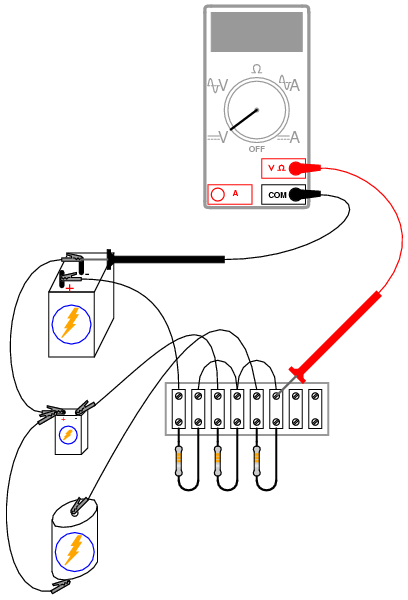3.15: A Very Simple Computer

PARTS AND MATERIALS

• Three batteries, each one with a different voltage
• Three equal-value resistors, between 10 kΩ and 47 kΩ each

When selecting resistors, measure each one with an ohmmeter and choose three that are the closest in value to each other. Precision is very important for this experiment!

CROSS-REFERENCES

Lessons In Electric Circuits, Volume 1, chapter 10: “DC Network Analysis”

LEARNING OBJECTIVES

• How a resistor network can function as a voltage signal averager
• Application of Millman’s Theorem

SCHEMATIC DIAGRAMILLUSTRATIONINSTRUCTIONS

This deceptively crude circuit performs the function of mathematically averaging three voltage signals together and so fulfills a specialized computational role. In other words, it is a computer that can only do one mathematical operation: averaging three quantities together.

Build this circuit as shown and measure all battery voltages with a voltmeter. Write these voltage figures on paper and average them together (E1 + E2 + E3, divided by three). When you measure each battery voltage, keep the black test probe connected to the “ground” point (the side of the battery directly joined to the other batteries by jumper wires), and touch the red probe to the other battery terminal. Polarity is important here! You will notice one battery in the schematic diagram connected “backward” to the other two, negative side “up.” This battery’s voltage should read as a negative quantity when measured by a properly connected digital meter, the other batteries measuring positive.

When the voltmeter is connected to the circuit at the point shown in the schematic and illustrations, it should register the algebraic average of the three batteries’ voltages. If the resistor values are chosen to match each other very closely, the “output” voltage of this circuit should match the calculated average very closely as well.

If one battery is disconnected, the output voltage will equal the average voltage of the remaining batteries. If the jumper wires formerly connecting the removed battery to the average circuit are connected to each other, the circuit will average the two remaining voltages together with 0 volts, producing a smaller output signal:The sheer simplicity of this circuit deters most people from calling it a “computer,” but it undeniably performs the mathematical function of averaging. Not only does it perform this function, but it performs it much faster than any modern digital computer can! Digital computers, such as personal computers (PCs) and pushbutton calculators, perform mathematical operations in a series of discrete steps. Analog computers perform calculations in continuous fashion, exploiting Ohm’s and Kirchhoff’s Laws for an arithmetic purpose, the “answer” computed as fast as voltage propagates through the circuit (ideally, at the speed of light!).

With the addition of circuits called amplifiers, voltage signals in analog computer networks may be boosted and re-used in other networks to perform a wide variety of mathematical functions. Such analog computers excel at performing the calculus operations of numerical differentiation and integration, and as such may be used to simulate the behavior of complex mechanical, electrical, and even chemical systems. At one time, analog computers were the ultimate tool for engineering research, but since then have been largely supplanted by digital computer technology. Digital computers enjoy the advantage of performing mathematical operations with much better precision than analog computers, albeit at much slower theoretical speeds.

COMPUTER SIMULATION

Schematic with SPICE node numbers:Netlist (make a text file containing the following text, verbatim):With this SPICE netlist, we can force a digital computer to simulate an analog computer, which averages three numbers together. Obviously, we aren’t doing this for the practical task of averaging numbers, but rather to learn more about circuits and more about computer simulation of circuits!